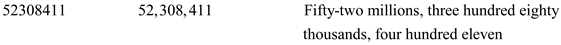# Quiz 1: Whole Numbers

Business

Steps for reading and writing whole numbers: Step 1: Beginning at the right side of the number, insert a comma every three digits to mark the groups Step 2: Beginning from left to right, name the digits and groups that have all zeros are not named. Step 3: When writing whole numbers in the word form, the numbers from 21 to 99 are hyphenated, expect for the decades (e.g., thirty). For example 83 would be written eighty-three. Note: The word "and" should not be used in reading or writing whole numbers. It represents the decimal point. Let the given number is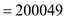Numerical form of the number is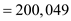Word form of the given number is Tw0-hundred thousand, forty-nine.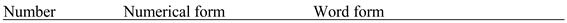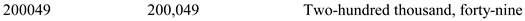The number system most widely used in the world today is known as the Hindu-Arabic numeral system, or decimal number system. The answer is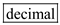Steps for reading and writing whole numbers: Step 1: Beginning at the right side of the number, insert a comma every three digits to mark the groups Step 2: Beginning from left to right, name the digits and groups that have all zeros are not named. Step 3: When writing whole numbers in the word form, the numbers from 21 to 99 are hyphenated, expect for the decades (e.g., thirty). For example 83 would be written eighty-three. Note: The word "and" should not be used in reading or writing whole numbers. It represents the decimal point. Let the given number is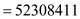Numerical form of the number is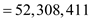Word form of the given number is Fifty-two million, three-hundred eight thousand, four hundred eleven.